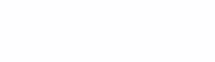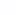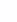# Autoencoders to remove noise from images##### Article author: Victoria Lyalikova

Getting deeper into machine learning and deep learning, I became very interested in the topic of autoencoders, especially in terms of noise removal. The search for various information of interest to me yielded results, but, unfortunately, almost everywhere the work of autoencoders is considered using the example of a very popular MNIST dataset. The collection of images of this set has a size of 28×28, the data is divided into sets for training and testing. However, I would like to see how autoencoders work in practice on more realistic images. And before we get started, let’s first take a look at what autoencoders are.

Autoencoders are special artificial neural network architectures that are trained to replicate their inputs into their outputs. Suppose we have an image, the autoencoder first encodes this image into a lower dimensional representation and then decodes the representation back into an image.

As you can see from the figure, the autoencoder has 3 main components:

1. Encoder or encoder – compresses data into a lower dimensional representation.

2. The code is the internal hidden layer, the part of the architecture that represents the compressed data, which is then sent to the decoder.

3. Decoder – decompresses or restores data from low and view to its original size.

In order to create an autoencoder model, two different functions are needed: an encoder and a decoder. Typically, these two functions are implemented using neural networks, and often when working with images, convolutional neural networks are usually used, and when working, for example, with text, LSTM networks can be used.

Now let’s turn to mathematics. The encoder translates the input signal into its representation (code) of the form h=g(x)Where x is our input vector, g – encoder. Next, the decoder restores the signal by its code x=f(h), f – decoder. The task of the autoencoder is to minimize the error functional L(x, f(g(x)). In this case, the families of functions g And f are limited so that the autoencoder is forced to select the most important properties of the signal.

In order to start training the autoencoder, you first need to define the following parameters:

1. Code size. The smaller the code size, the greater the compression.

2. The number of layers in the encoder and decoder. Layer depths can reach any level.

3. The number of nodes in the level. Typically, the number of nodes per level decreases with each successive encoder level, and then begins to increase again with each successive decoder level. Those. the decoder is symmetrical to the structure of the encoder, but this is not a requirement.

4. Loss function. The most popular options are root mean square error (MSE) or binary cross entropy.

To model neural networks in python, it is very convenient to use the high-level Keras library, which is a wrapper over Tensorflow.

In Keras, to build neural network models (models), we collect layers (layers). To describe the standard architectures of neural networks in Keras, there are already predefined classes for layers:

• Dense() is a fully connected layer;

• Conv1D, Conv2D, Conv3D – convolutional layers;

• Conv2DTranspose, Conv3DTranspose – transposed (inverse) convolutional layers;

• SimpleRNN, LSTM, GRU – recurrent layers;

• MaxPooling2D, UpSampling2D, Dropout, BatchNormalization – auxiliary layers

Now you can start building an autoencoder for image noise reduction.

To implement this task, we will use a dataset containing MRI images of the brain. First, let’s load the required libraries:

import imutils
import cv2
import os
from imutils import paths
import numpy as np
from PIL import Image
import matplotlib.pyplot as plt
from keras.layers import Input, Conv2D, MaxPool2D, UpSampling2D
from keras.models import Model
from sklearn.model_selection import train_test_split

The data is divided into 2 folders, one folder contains images that do not have anomalies, and the second contains images with brain tumors. There are 253 images in total. For now, let’s load 1 image from each folder and look at them ..

image=cv2.imread('D:/*****/brain_tumor_dataset/no/23 no.jpg')
plt.imshow(image)

We see that the height of the first image is 338, the width is 276, and the height of the second image is 201, the width is 173, the color channels are 3.

Now our task is to add noise to the images, and then feed the noisy images to the input of the autoencoder and compare the output with data without noise.

Now you can start working with the data. First, we list the contents of the “no” and “yes” directories using the os.listdir() command. Next, using the OpenCV library and the cv2.imread() command, we will read our image into a three-dimensional array.

Firstly, all images are of different sizes, which means that the size must be somehow unified, and secondly, it is necessary to convert the matrix representations of the image into a vector [0,1]. We will reduce all images to the size 256×256, and then we will divide each pixel value by 255.

img_r =256
folder_path = "D:/*******/brain_tumor_dataset"
no_images = os.listdir(folder_path + '/no/')
yes_images = os.listdir(folder_path + '/yes/')
dataset=[]
for image_name in no_images:
image=Image.fromarray(image)
image=image.resize((img_r ,img_r ))
image2arr = np.array(image)/255
dataset.append(image2arr)

for image_name in yes_images:
#image = image+noise2
image=Image.fromarray(image)
image=image.resize((img_r ,img_r ))
image2arr = np.array(image)/255
dataset.append(image2arr) 

Now we will divide the entire data set into training and test samples, and then we will add noise to these data.

X_train, X_test = train_test_split(dataset, test_size= 0.25, random_state = 42)

First with the library numpy Let’s model a Gaussian noise with zero mathematical expectation and a standard deviation equal to one, and then add it to the training and test set with a coefficient of 0.4.

noise =  np.random.normal(loc=0, scale=1, size=(img_r,img_r,1))
x_train_noise = np.clip((np.array(X_train)+noise*0.4),0,1)
x_test_noise = np.clip((np.array(X_test)+noise*0.4),0,1)

Now we can proceed to the architecture of the neural network. The size of the input layer is equal to the size of the image (256, 256.3). Due to the fact that our data is images, then we will create an autoencoder consisting of convolutional layers. As mentioned above, we need to create two functions: an encoder and a decoder. The encoder will consist of two convolutional layers 256x3x3 and 128x3x3, respectively, and two layers with a maximum pooling of 2×2.

# input layer
input_layer = Input(shape=(img_r,img_r,3))
#encoder
encoded_layer1 = Conv2D(256, (3, 3), activation='relu', padding='same')(input_layer)
encoded_layer1 = MaxPool2D( (2, 2), padding='same')(encoded_layer1)
encoded_layer2 = Conv2D(128, (3, 3), activation='relu', padding='same')(encoded_layer1)
encoded_layer2 = MaxPool2D( (2, 2), padding='same')(encoded_layer2)
encoded = Conv2D(64, (3, 3), activation='relu', padding='same')(encoded_layer2)

A decoder is essentially the exact opposite of an encoder, as we are restoring a 2D representation of our images. It will consist of two 128x3x3 and 256x3x3 convolutional layers, respectively, and two 2×2 upsampling layers.

decoded_layer1 = Conv2D(128, (3, 3), activation='relu', padding='same')(encoded)
decoded_layer1 = UpSampling2D((2, 2))(decoded_layer1)
decoded_layer2 = Conv2D(256, (3, 3), activation='relu', padding='same')(decoded_layer1)
decoded_layer2 = UpSampling2D((2, 2))(decoded_layer2)
output_layer   = Conv2D(3, (3, 3), padding='same', activation='sigmoid')(decoded_layer2)

All but the last convolutional layer uses Relu’s linear activation function, since we’re dealing with pixel values. The last layer of the decoder uses a sigmoidal activation function.

We connect the input and output to form and compile the autoencoder. We will use the mean square error as the loss function. We visualize our network using the model.summary() function.

# compile the model
model = Model(input_layer, output_layer)
model.summary()

Now we can start training our model.

history = model.fit(x_train_noise, x_train, epochs=50, validation_data=(x_test_noise, x_test)).

As we can see, the encoder receives x_train_noise noisy images as input and learns to compress them, and then the decoder learns to decompress them into clean images without noise.

Let’s look at the loss graphs during the training and verification stages.

plt.plot(history.history['loss'])
plt.plot(history.history['val_loss'])
plt.title('Потери на этапах проверки и обучения')
plt.ylabel('Потери')
plt.xlabel('Эпохи')
plt.legend(['Потери на этапе обучения', 'Потери на этапе проверки'], loc="upper left")
plt.show()

We see that even after 50 epochs, the network is slowly but still learning, the losses at the verification stages also fall, as well as at the training stages. After training the neural network, you can save the autoencoder weights for further use.

json_string = model.to_json()
model.save_weights('autoencoder.h5')
open('autoencoder_N_04_50.h5','w').write(json_string)

Now you can visualize the received data. Let’s look at the first 5 original images, noisy images, and autoencoder reconstructed images with different numbers of training epochs.

n = 5
plt.figure(figsize=(20, 20))

for i in range(n):
# оригинальные изображения
ax = plt.subplot(3, n, i + 1)
plt.imshow((x_test[i]))
plt.gray()
ax.get_xaxis().set_visible(False)
ax.get_yaxis().set_visible(False)

# зашумленные изображения
ax = plt.subplot(3, n, i + 1 + n)
plt.imshow(x_test_noise[i])
plt.gray()
ax.get_xaxis().set_visible(False)
ax.get_yaxis().set_visible(False)

# восстановленные изображения автоэнкодером
ax = plt.subplot(3, n, i + 1 + 2*n)
plt.imshow(np.array(decoded_imgs[i]))
plt.gray()
ax.get_xaxis().set_visible(False)
ax.get_yaxis().set_visible(False)
plt.show()

It can be seen that with an increase in the number of training epochs, the autoencoder learns to restore images more efficiently. Next, we quantify the accuracy of the network using the root mean square error RMSE – the root of the mean squared errors according to the formulaWhere– real values,– predicted values

lab_err = []
for i in range(5):
pred = np.array(decoded_imgs[i])
target = x_test[i]
err = np.sqrt(np.mean((target-pred)**2))
lab_err.append(err)
print("Image error:",lab_err,'\n')

RMSE for 10 training epochs

Image error: [0.11112235583367339, 0.09649739151342915, 0.07507075125156328, 0.09451597002239683, 0.11461512072947239]

RMSE for 50 training epochs

Image error: [0.077847916992295, 0.06904349103850838, 0.052240344819613975, 0.04785264086429222, 0.0692672959161245]

And, in principle, it is noticeable that with an increase in the number of training epochs, the error decreases. I think that for the sake of clarity of the experiment, for the time being, we can dwell on 50 learning epochs. You can only try to add noise to the original images with a smaller coefficient equal to 0.2 and also train the autoencoder for 50 epochs, and then calculate the RMSE.

RMSE after 50 training epochs

Image error: [0.0650505273762427, 0.05470585227284887, 0.04235355301246957, 0.03651446513302648, 0.05535199588180513]

As expected, the RMSE value is smaller for images with a noise factor of 0.2 than for images with a noise factor of 0.4. It is easier for the autoencoder to recover less noisy images.

And finally, I wanted to conduct an experiment to restore noisy color images. For this, a dataset containing images of forests and sea coasts was chosen. The architecture of the neural network has remained the same, so I will present here only the results obtained.